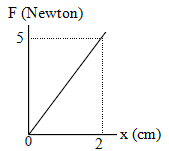# Potential energy of elastic spring – problems and solutions

1. A 2-kg mass is attached to a spring. If the elongation of spring is 4 cm, determine potential energy of elastic spring. Acceleration due to gravity is 10 m/s2.

Known :

Mass (m) = 2 kg

Acceleration due to gravity (g) = 10 m/s2

Weight (w) = m g = (2)(10) = 20 N

Elongation (x) = 4 cm = 0.04 m

Wanted : Potential energy of elastic spring

Solution :

Formula of Hooke’s law :

F = k x

F = force, k = spring constant, x = the change in length of spring

Spring constant :

k = w / x = 20 / 0.04 = 500 N/m

Potential energy of elastic spring :

PE = ½ k x2 = ½ (500)(0.04)2 = (250)(0.0016) = 0.4 Joule

Alternative solution :

PE = ½ k x2 = ½ (w / x) x2 = ½ w x = ½ m g x

w = weight, m = mass, x = the change in length of spring

PE = ½ (2)(10)(0.04) = (10)(0.04) = 0.4 Joule.

[irp]

2. The elongation of spring stretched by a force F = 50 N is 10 cm. What is the potential energy of elastic spring if the elongation of spring is 12 cm.

Known :

Elongation (x) = 10 cm = 0.1 m

Force (F) = 50 N

Wanted : The potential energy of elastic spring

Solution :

Spring constant :

k = F / x = 50 / 0.1 = 500 N/m

The potential energy of elastic spring if the elongation of spring is 12 cm. :

PE = ½ k x2 = ½ (500)(0.12)2 = (250)(0.0144) = 3.6 Joule.

[irp]

3. Graph of F vs x shown in figure below. What is the potential energy of elastic spring, if the elongation of spring is 10 cm.Known :

Force (F) = 5 N

The elongation of spring (x) = 2 cm = 0.02 m

Wanted: The potential energy of elastic spring if the elongation of spring is 0.1 m.

Solution :

Spring constant :

k = F / x = 5 / 0.02 = 250 N/m

The potential energy of elastic spring if the elongation of spring is 0.1 m :

PE = ½ k x2 = ½ (250)(0.1)2 = (125)(0.01) = 1.25 Joule.

4. An athlete jumps onto a spring instrument with a weight of 500 N, the spring shortens 4 cm. Determine the amount of the potential energy to force the athlete.

A. 20 Joule

B. 10 Joule

C. 5 Joule

D. 2 Joule

Known :

Force (F) = 500 N

The change in length of spring (Δx) = 4 cm = 0.04 m

Wanted: The potential energy of spring

Solution :

Calculate the elastic constant of a spring using the equation of Hooke’s law :

k = F / Δx = 500 N / 0.04 m = 12500 N/m

The elastic potential energy of spring :

PE = ½ k Δx2 = ½ (12500)(0.04)2 = (6250)(0.0016)

PE = 10 Joule

5. A spring when suspended with a mass of 500 grams, its length increases by 5 cm. Determine its constant and its potential energy.

Known :

Mass of object (m) = 500 gram = (500/1000) kg = 5/10 kg = 0.5 kg

Acceleration (g) = 10 m/s2

Object weight (w) = m g = (0.5 kg)(10 m/s2) = 5 kg m/s2 = 5 Newton

The change in length of spring (Δx) = 5 cm = 0.05 m

Wanted : spring’s constant (k) and potential energy (PE)

Solution :

First, calculate spring’s constant using equation of Hooke’s law :

k = w / Δx = 5 N / 0.05 m = 100 N/m

The potential elastic of spring :

PE = ½ k Δx2 = ½ (100)(0.05)2 = (50)(0.0025)

PE = 0.125 Joule

[wpdm_package id=’1179′]